# Strength of materials

 Strength of materials deals with the effect of forces on deformable bodies. In addition, material-dependent parameters should be considered as well. An introduction to the strength of materials is, therefore, given by the concept of stress and strain and by Hooke’s law, which is applied to tension, pressure, torsion and bending problems.
Elastic deformations
 The load from external forces causes stresses in the components. The mesh of the material is deformed under the action of a force, e.g. compressed, stretched etc. Elastic deformation means the atoms return to their original position after the action of the force has ceased.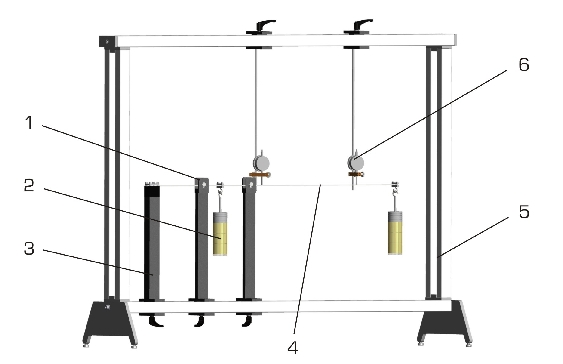SE 110.14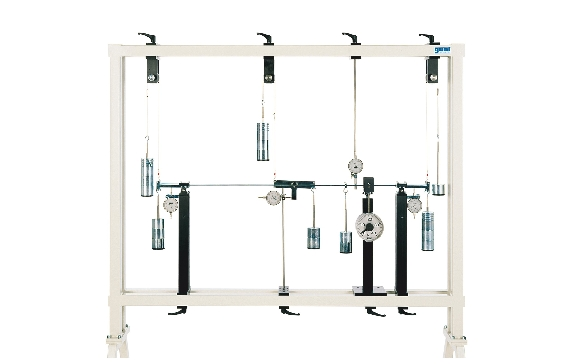SE 110.47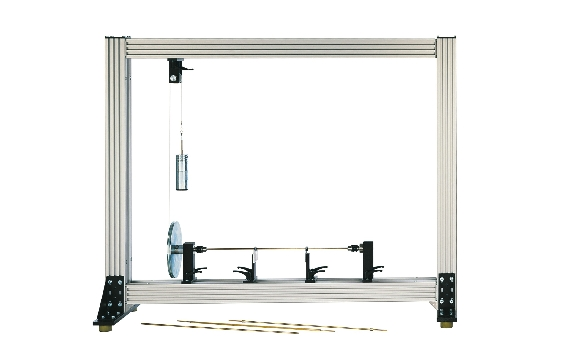SE 110.29 Elastic line of a beam Methods to determine the elastic line Torsion of bars Demonstration of Maxwell-Betti theorem Determination of elastic lines of a beam under load using the principle of virtual work and Mohr’s Analogy Investigation of elastic torsion of bars with open and closed cross-section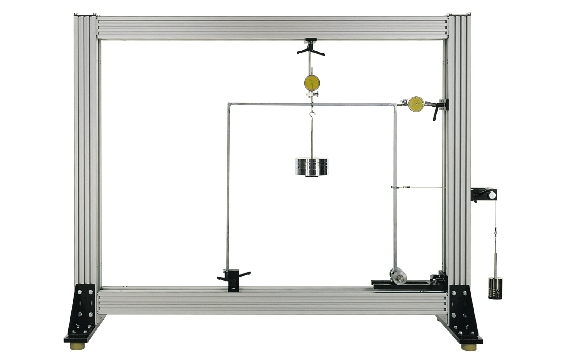SE 110.20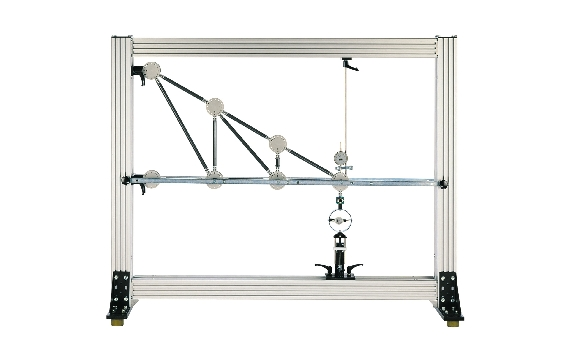SE 110.44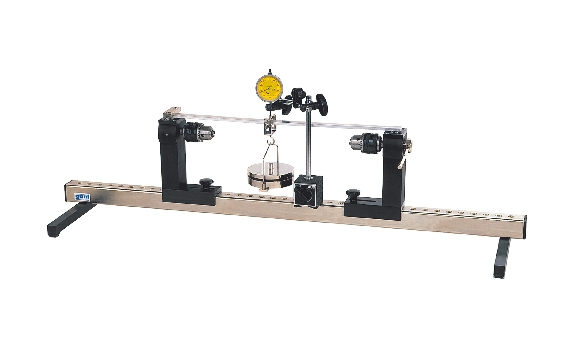WP 100 Deformation of frames Deformation of trusses Deformation of bars under bending or torsion Elastic deformation of a statically determinate or overdeterminate frame under point load Application of Castigliano’s first theorem Influence of material, cross-section and clamping length on deformation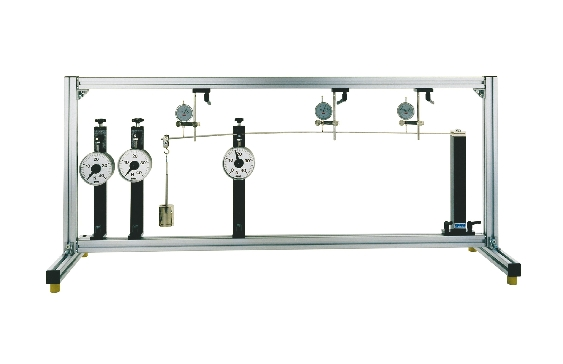WP 950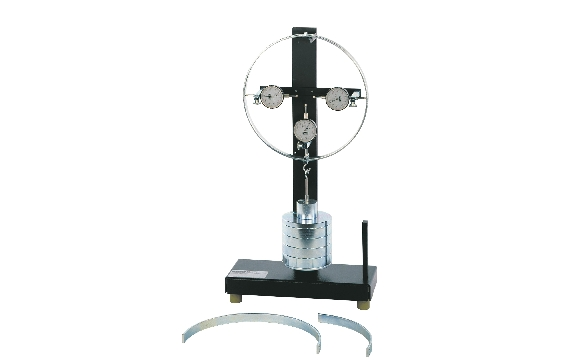FL 170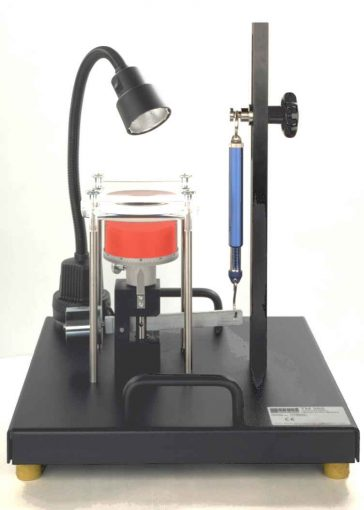TM 262 Deformation of straight beams Deformation of curved-axis beams Hertzian pressure Elastic lines of statically determinate and overdeterminate beams under various clamping conditions Principle of virtual forces for calculating deformation Demonstration of the resulting characteristics of the contact area as a function of the contact force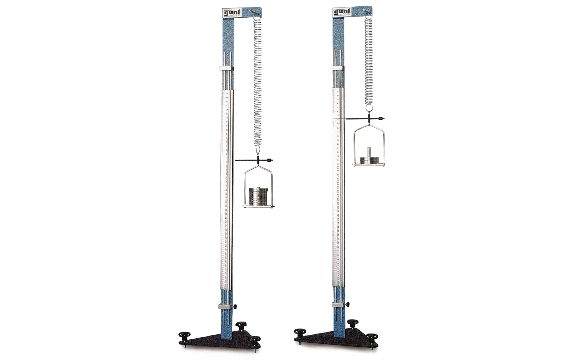TM 400 Hooke’s law Elastic behaviour of tension springs under load
Buckling and stability

Long and slender components such as rods, beams, supports etc. may end up in indifferent or unstable equilibrium states and buckle if they are exposed to a force along the rod axis under compressive stress. The mathematician and physicist Leonhard Euler defined four typical buckling cases to calculate the buckling force in rods.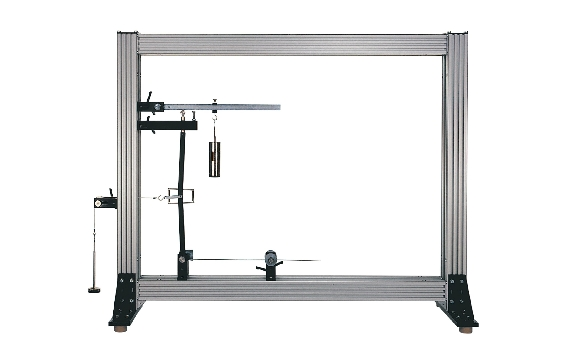SE 110.19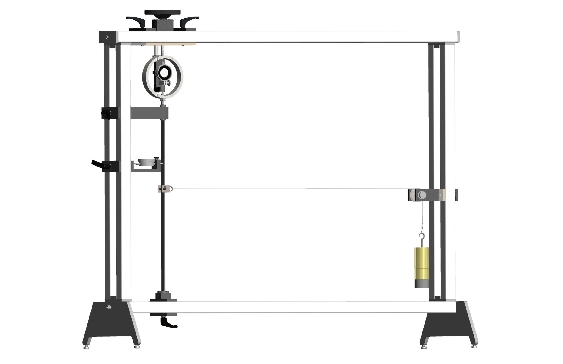SE 110.57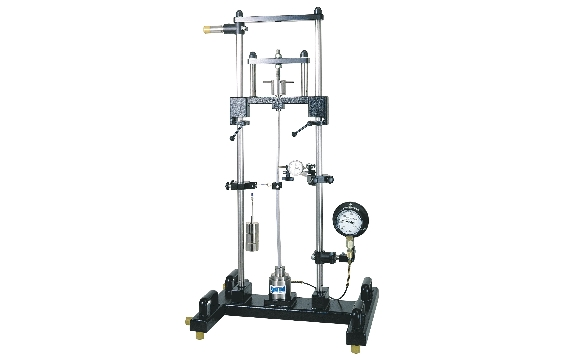WP 120 Investigation of simple stability problems Buckling of bars Buckling behaviour of bars Determination of the buckling load under different conditions SE 112 Mounting Frame required Determination of the buckling load: influence of material, support, and shear force Correlation between buckling length, buckling load and various methods of support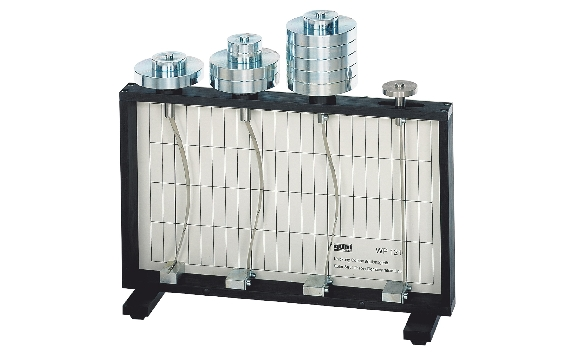WP 121 Demonstration of Euler buckling Verification of the Euler theory of buckling and buckling behaviour under the influence of material, cross-section, length, and support
Compound stress

Experimental equipment to investigate components in which two or more basic stresses are present simultaneously.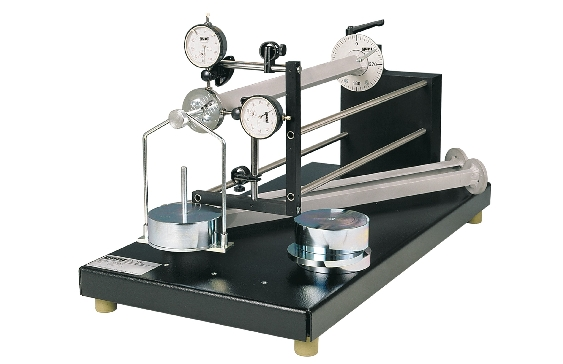FL 160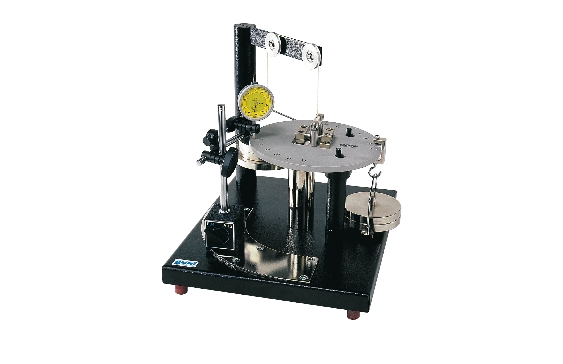WP 130 Unsymmetrical bending Verification of stress hypotheses Investigation of symmetrical and unsymmetrical bending on a beam. Combined bending and torsion loading using an eccentric force. Multiaxial loading of samples by bending and torsion
Experimental stress and strain analysis
 Two methods of non-destructive experimental stress and strain analysis are presented here: - the electrical method of strain measurement using strain gauges to indirectly determine the actual stresses - the photoelastic method for a direct representation of the stress distribution.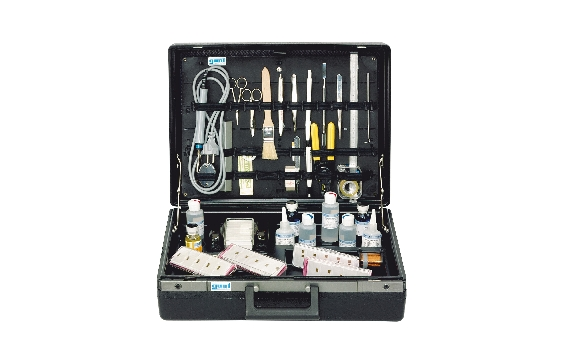FL 101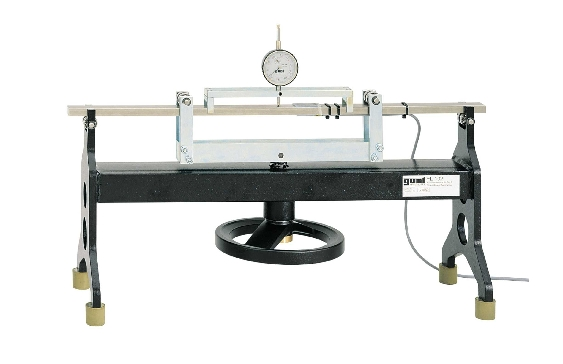FL 102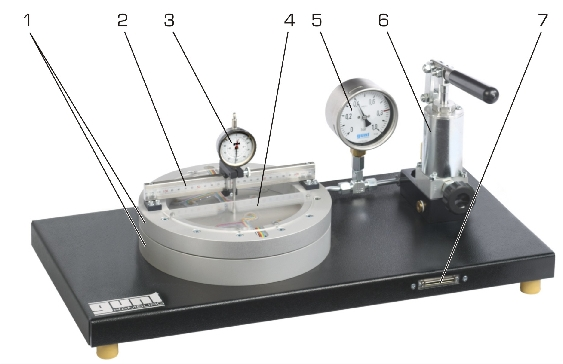FL 120 Strain gauge application set Determining the gauge factor of strain gauges Stress and strain analysis on a membrane Complete equipment for practising manual handling of strain gauge technology Basic introduction to measurement with strain gauges for tension, bending and torsion Investigation of deflection and strain to determine the gauge factor of strain gauges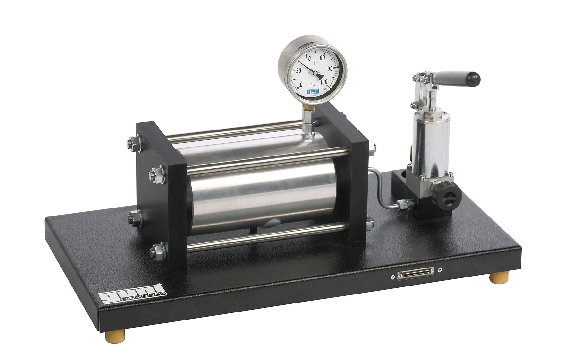FL 140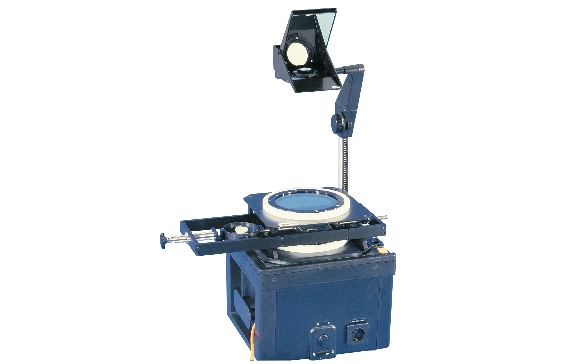FL 210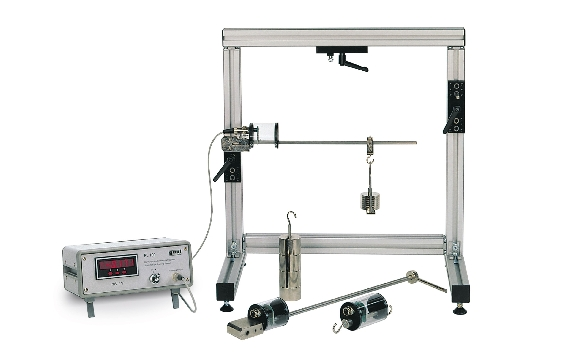FL 100 Stress and strain analysis on a thick-walled cylinder Photoelastic demonstration Strain gauge training system Triaxial stress state in the cylinder wall; cylinder with strain gauge application on surface and in wall Representation of distribution of stress and stress concentrations in component models. Can be used in conjunction with an overhead projector. Basic introduction to measurement with strain gauges for tension, bending and torsion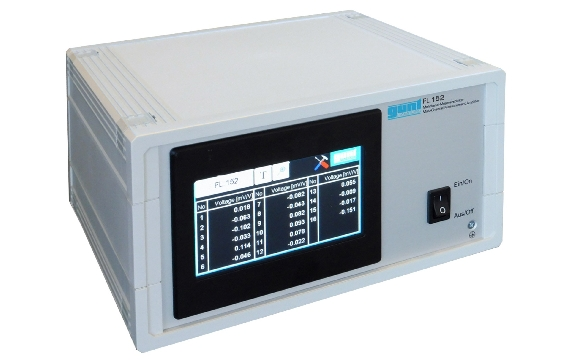FL 152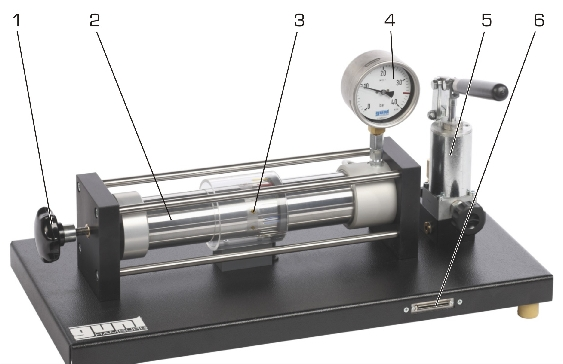FL 130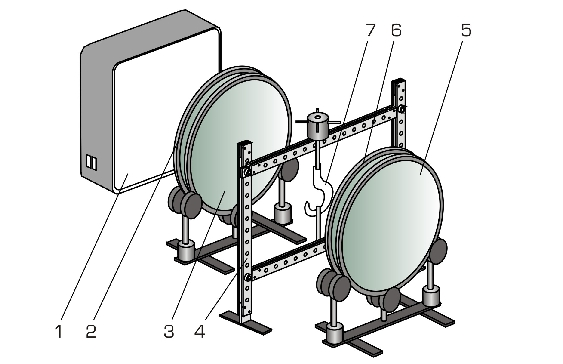FL 200 Multi-channel measuring amplifier Stress and strain analysis on a thin-walled cylinder Photoelastic experiments with a transmission polariscope Processing of analogue measuring signals for FL 120 – FL 140 and for GUNT trusses Investigation of axial and circumferential stress in a thin-walled cylinder under internal pressure Visualisation of mechanical stresses in models subject to varying loads